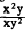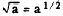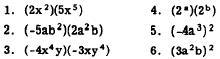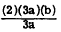Exponents and radicalsCustom SearchEXPONENTS AND RADICALS Exponents and radicals have the same meaning in algebra as they do in arithmetic. Thus, if n represents any number then n2 = n · n, n3 = n · n · n, etc. By the same reasoning, nm means that n is to be taken as a factor m times. That is, nm is equal to n · n · n . . . ..with n appearing m times. The series of dots, called ellipsis (not to be confused with the geometric figure having a similar name, ellipse), represents continuation of the same pattern or the same symbol. The rules of operation with exponents are also the same in algebra as in arithmetic. For example, n2 · . Some care is exponents is a number or a letter. Thus the general form can be expressed as follows: na .  nb = na+b In words, the general rule for multiplication involving exponents is as follows: When multiplying terms whose literal factors are like, the exponents are added. This rule may be applied to problems involving division, if all expressions containing exponents in denominators are rewritten as expressions with negative exponents. For example, the fractioncan be rewritten as (x2y)(x-1y-2), which is equal to (x2-1)(y1-2). This reduces to xy-1, or x/y Notice that the result is the same as it would have been if we had simply subtracted the exponents of literal factors in the denominator from the exponents of the same literal factors in the numerator. The algebraic rules for radicals also remain the same as those of arithmetic. In arithmetic,Likewise, in algebraandMULTIPLYING MONOMIALS If a monomial such as 3abc is to be multiplied by a numerical multiplier, for the coefficient alone is multiplied, following example: 5 x 3abc = 15abc When the numerical factor is not the initial factor of the expression, as in x(2a), the result of the multiplication is not written as x2a. Instead, the numerical factor is interchanged with literal factors by use of the commutative law of multiplication. The literal factors are usually interchanged to place them in alphabetical order, and the final result is as follows:     x(2a) = 2ax The rule for multiplication of monomials may be stated as follows: Multiply the numerical coefficients to form the coefficient of the product. Multiply the literal factors, combining exponents in like factors, to form the literal part of the product. The complete process is illustrated in the following example: (2ab)(3a2)(2b3) = 12a1+2b1+3                             = 12a3b4 Practice problems. Perform the indicated operations:Answers:DIVIDING MONOMIALS As may be expected, the process of dividing is the inverse of multiplying. Because 3 x 2a = 6a, 6a ¸ 3 = 2a, or 6a ¸ 2 = 3a. Thus, when the divisor is numerical, divide the coefficient of the dividend by the divisor. When the divisor contains literal parts that are also in the dividend, cancellation may be performed as in arithmetic. For example, 6ab + 3a may be written as follows:Cancellation of the common literal factor, 3a, from the numerator and denominator leaves 2b as the answer for this division problem. When the same literal factors appear in both the divisor and the dividend, but with different exponents, cancellation may still be used, .as follows:This same problem may be solved without thinking in terms of cancellation, by rewriting with negative exponents as follows:Practice problems. Perform the indicated operations:Integrated Publishing, Inc. - A (SDVOSB) Service Disabled Veteran Owned Small Business